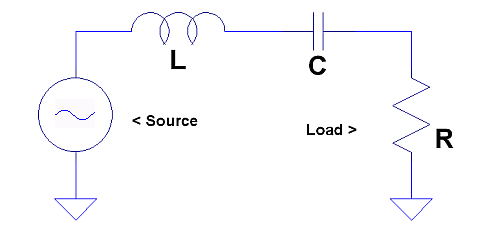## Series Resonant Filter Values Calculator

### Calculates inductance (L) and capacitance (C) values for a specified operating center frequency, load resistance and Q.

Enter center frequency:

*The calculator assumes negligible source resistance. If the source resistance is known, enter the sum of load resistance and source resistance. Note also that coil resistance and capacitor equivalent series resistance may be significant. The Q performance of the working circuit will depend on the total of source, load, coil and capacitor resistances.

Enter desired circuit Q:
Note: The filter's Q value is the inverse of its -3 dB bandwidth.
See frequency response plots.

back

close

more calculators

maxmcarter.comX = QR L = X/(2πF) C = 1/(2πXF)